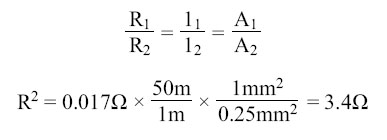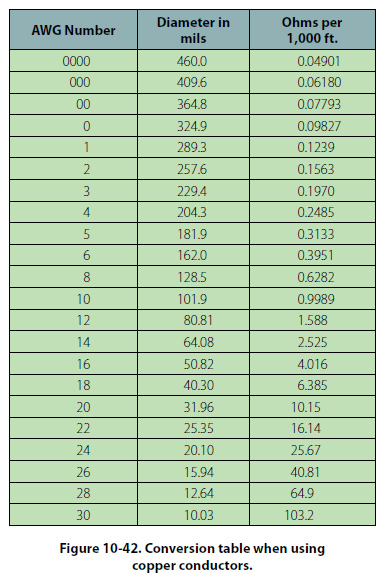Resistance and Its Relation to Wire Sizing Circular Conductors (Wires/Cables) Because it is known that the resistance of a conductor is directly proportional to its length, and if we are given the resistance of the unit length of wire, we can readily calculate the resistance of any length of wire of that particular material having the same diameter. Also, because it is known that the resistance of a conductor is inversely proportional to its cross-sectional area, and if we are given the resistance of a length of wire with unit cross-sectional area, we can calculate the resistance of a similar length of wire of the same material with any cross-sectional area. Therefore, if we know the resistance of a given conductor, we can calculate the resistance for any conductor of the same material at the same temperature. From the relationship: R = (ρ × l)/A It can also be written: R1/R2 = l1/l2 = A1/A2While the System International (SI) units are commonly used in the analysis of electric circuits, electrical conductors in North America are still being manufactured using the foot as the unit length and the mil (one thousandth of an inch) as the unit of diameter. Before using the equation R = (ρ × l)/A to calculate the resistance of a conductor of a given AWG size, the crosssectional area in square meters must be determined using the conversion factor 1 mil = 0.0254 mm. The most convenient unit of wire length is the foot. Using these standards, the unit of size is the mil-foot. Thus, a wire has unit size if it has a diameter of 1 mil and length of 1 foot. In the case of using copper conductors, we are spared the task of tedious calculations by using a table as shown in Figure 10-42.Note that cross-sectional dimensions listed on the table are such that each decrease of one gauge number equals a 25 percent increase in the cross-sectional area. Because of this, a decrease of three gauge numbers represents an increase in cross-sectional area of approximately a 2:1 increase. Likewise, change of ten wire gauge numbers represents a 10:1 change in cross-sectional area — also, by doubling the cross-sectional area of the conductor, the resistance is cut in half. A decrease of three wire gauge numbers cuts the resistance of the conductor of a given length in half.
 ©AvStop Online Magazine                                                                                                                                                      Contact Us              Return To Books